NR UCI Multiplexing on PUSCH

This example shows the different processing steps involved in the data and control multiplexing to form a codeword associated with a physical uplink shared channel (PUSCH) using 5G Toolbox™ features.

Introduction

Uplink control information (UCI) messages consist of a hybrid automatic repeat request acknowledgment (HARQ-ACK), channel state information (CSI), and a scheduling request (SR). These UCI messages are encoded and transmitted through the physical uplink control channel (PUCCH) or are multiplexed on the PUSCH. The CSI reporting configuration can be aperiodic (using a PUSCH), periodic (using a PUCCH), or semi-persistent (using a PUCCH or DCI-activated PUSCH). A CSI report comprises of two parts. CSI part 1 has a fixed payload size and is used to identify the number of information bits in CSI part 2. CSI part 1 must be transmitted completely before the transmission of CSI part 2. The HARQ-ACK (if any) and CSI (if any) is encoded and multiplexed with or without encoded UL-SCH data, and then transmitted on a PUSCH.

Data and Control Multiplexing

The encoded data, encoded HARQ-ACK, encoded CSI part 1, and encoded CSI part 2 are multiplexed to form a codeword with the steps outlined in TS 38.212 Section 6.2.7 .

The UCI information is transmitted in only the OFDM symbols that are unused for demodulation reference signal (DM-RS) transmission. In any OFDM symbol used for UCI transmission for a UCI type, the mapping of that UCI type depends on the number of resource elements (REs) available for UCI transmission and the remaining REs required for that UCI type. If the number of remaining REs required for that UCI type in an OFDM symbol is greater than half of the available REs for the UCI transmission, the mapping of the UCI type is contiguous. Otherwise, the mapping is uniformly distributed across available REs in an OFDM symbol to achieve the diversity gain. The number of coded bits that are occupied in an RE for UCI or data transmission, is equal to the product of the modulation order and the number of layers.

The coded HARQ-ACK bits are placed from the OFDM symbol, after the first consecutive DM-RS OFDM symbols. The coded CSI part 1 or part 2 bits are placed at the starting OFDM symbol that is unused for DM-RS in the shared channel symbol allocation. The multiplexing operation depends on the number of HARQ-ACK bits. When the number of HARQ-ACK bits is less than or equal to 2, the coded HARQ-ACK bits are punctured. Otherwise, the coded HARQ-ACK bits are rate-matched.

Multiplexing involves these processing steps.

• Step 1: When the number of HARQ-ACK bits is less than or equal to 2, find the reserved HARQ-ACK locations.

• Step 2: When the number of HARQ-ACK bits is greater than 2, map the coded HARQ-ACK bits (if any).

• Step 3: Map the coded CSI part 1 and CSI part 2 bits (if any).

• Step 4: Map the coded UL-SCH bits (if any).

• Step 5: When the number of HARQ-ACK bits is less than or equal to 2, map the coded HARQ-ACK bits (if any).

• Step 6: Form the codeword.

This example shows steps 1 to 6 for two separate cases for the PUSCH, with one resource block occupying all of the OFDM symbols in a slot, a single layer, a pi/2-BPSK modulation scheme, and DM-RS symbols occupying OFDM symbols 2, 7, and 11 (0-based). The REs other than DM-RS REs in the DM-RS OFDM symbols are used for data transmission. For the first case, the number of HARQ-ACK bits is less than or equal to 2. For the second case, the number of HARQ-ACK bits is greater than 2. This figure shows the grid with only the DM-RS symbol locations. This grid is populated with the coded types UL-SCH, HARQ-ACK, CSI part 1, and CSI part 2, according to the multiplexing operation for each case. The transport block size is set to 24, and the target code rate is set to 314/1024. The number of payload bits of each CSI part is set to 10, and all of the associated beta factors are set to 1.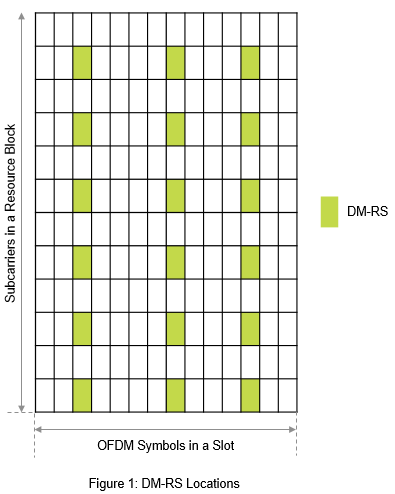Configure Carrier Resource Grid and PUSCH

Configure an nrCarrierConfig object to create a 15 kHz carrier resource grid with one resource block. Configure an nrPUSCHConfig object to get the respective DM-RS locations as shown in the previous figure. Also, configure the UCI parameters (BetaOffsetACK, BetaOffsetCSI1, BetaOffsetCSI2, and UCIScaling). The property BetaOffsetACK determines the number of resources for multiplexing HARQ-ACK in a PUSCH. The properties BetaOffsetCSI1 and BetaOffsetCSI2 determine the number of resources for multiplexing CSI reports in a PUSCH. The property UCIScaling limits the number of REs assigned to the UCI on PUSCH.

% Set the carrier configuration
carrier = nrCarrierConfig;
carrier.SubcarrierSpacing = 15;   % Subcarrier spacing in kHz (15, 30, 60, 120, 240)
carrier.CyclicPrefix = 'normal';  % Cyclic prefix ('normal' or 'extended')
carrier.NSizeGrid = 1;            % Number of resource blocks in carrier resource grid (1...275)

% Set the PUSCH configuration
pusch = nrPUSCHConfig;
pusch.Modulation = 'pi/2-BPSK';     % Modulation scheme ('pi/2-BPSK', 'QPSK', '16QAM', '64QAM', or '256QAM')
pusch.NumLayers = 1;                % Number of layers (1, 2, 3, or 4)
pusch.SymbolAllocation = [0 14];    % OFDM symbol allocation [S L]
pusch.PRBSet = 0;                   % Vector of PRB allocated with values in the range [0, 274]
pusch.MappingType = 'A';            % Mapping type ('A' or 'B')
pusch.FrequencyHopping = 'neither'; % Frequency hopping configuration ('neither', 'interSlot', or 'intraSlot')
pusch.SecondHopStartPRB = 0;        % Second hop start PRB in the range [0, 274], ensure the combination with PRBSet does not exceed 274
pusch.TransformPrecoding = 0;       % Transform precoding (0 or 1)

% Set the UCI parameters
pusch.BetaOffsetACK = 1;  % Beta offset for HARQ-ACK
pusch.BetaOffsetCSI1 = 1; % Beta offset for CSI part 1
pusch.BetaOffsetCSI2 = 1; % Beta offset for CSI part 2
pusch.UCIScaling = 1;     % UCI scaling factor

% Set the DM-RS parameters
pusch.DMRS.DMRSConfigurationType = 1;   % DM-RS configuration type (1 or 2)
pusch.DMRS.DMRSTypeAPosition = 2;       % DM-RS type A position (2 or 3)
pusch.DMRS.DMRSLength = 1;              % DM-RS length (1 or 2)
pusch.DMRS.DMRSAdditionalPosition = 2;  % Number of DM-RS additional positions (0, 1, 2, or 3)
pusch.DMRS.NumCDMGroupsWithoutData = 1; % Number of CDM groups without data (1, 2, or 3)

% Set the PT-RS parameters
pusch.EnablePTRS = 0;            % Disable or Enable PT-RS (0 or 1)
pusch.PTRS.FrequencyDensity = 2; % PT-RS frequency density (2 or 4)
pusch.PTRS.TimeDensity = 1;      % PT-RS time density (1, 2, or 4)
pusch.PTRS.REOffset = '00';      % PT-RS resource element offset ('00', '01', '10', or '11')
pusch.PTRS.NumPTRSSamples = 2;   % Number of PT-RS samples (2 or 4)
pusch.PTRS.NumPTRSGroups = 2;    % Number of PT-RS groups (2, 4, or 8)

% Set the target code rate, transport block size, payload lengths of CSI part 1 and CSI part 2
tcr = 314/1024; % Target code rate in the range (0, 1)
tbs = 24;       % Transport block size
ocsi1 = 10;     % Number of CSI part 1 bits
ocsi2 = 10;     % Number of CSI part 2 bits

Case 1: Number of HARQ-ACK Bits Less Than or Equal to 2

For illustration purposes, the number of HARQ-ACK bits is set to 1 in this example. To obtain the information about the number of coded bits of each type, use the nrULSCHInfo function. For the specified PUSCH configuration, target code rate, and payload values, the number of coded HARQ-ACK bits is 2, the number of coded CSI part 1 and CSI part 2 bits is 19 each, and the number of coded UL-SCH bits is 94.

Step 1: When the number of HARQ-ACK bits is less than or equal to 2, find the reserved HARQ-ACK locations and mark them on the grid.

The number of reserved HARQ-ACK locations is achieved by calculating the rate-matching length of the HARQ-ACK with number of HARQ-ACK bits set to 2. With the specified PUSCH configuration, target code rate, and transport block size, the number of reserved HARQ-ACK locations is 4. The HARQ-ACK is mapped to the REs in the OFDM symbol that is available after the first consecutive DM-RS OFDM symbols. This figure shows the locations of reserved HARQ-ACK.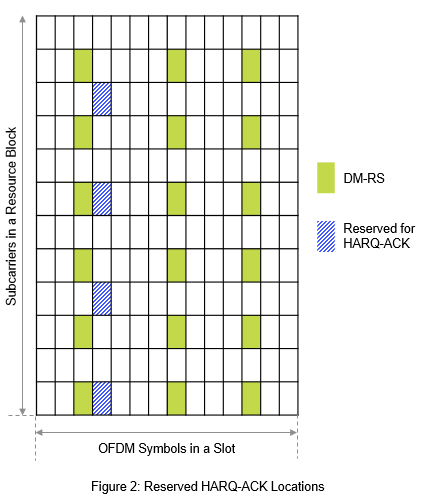Step 2: When the number of HARQ-ACK bits is greater than 2, map the coded HARQ-ACK bits.

Because the number of HARQ-ACK bits is less than or equal to 2 in this case, skip this step.

Step 3: Map the coded CSI part 1 and CSI part 2 bits

The CSI mapping (CSI part 1 followed by CSI part 2) starts from the first non-DMRS OFDM symbol available in the PUSCH allocation. For the configured setup, the mapping starts from OFDM symbol 0 (0-based). The mapping locations are determined based on the number of REs available and the number of REs required for CSI part 1 transmission. CSI part 1 transmission required 19 REs. Because only 12 REs are available for transmission, every RE is occupied in this case for CSI part 1 in OFDM symbol 0. For the transmission of the remaining CSI part 1 symbols, the mapping goes to the next OFDM symbol not used for DM-RS (that is, OFDM symbol 1). In OFDM symbol 1, 12 REs are available, but CSI part 1 requires only 7 REs. Because the remaining REs required for CSI part 1 are more than half of the REs available for UCI transmission, CSI part 1 is mapped to contiguous REs. This figure shows this scenario.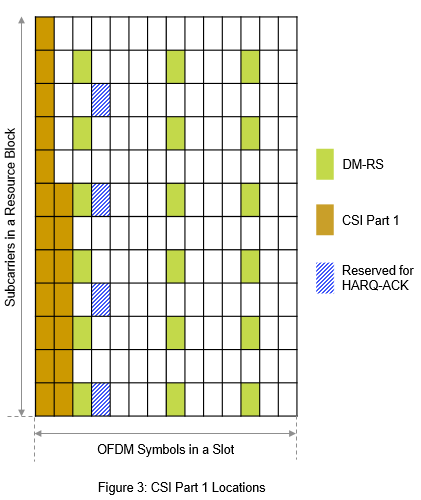Once the coded CSI part 1 is completely mapped, the mapping of coded CSI part 2 starts. The mapping starts from the first non-DMRS OFDM symbol that is used for PUSCH transmission (that is, OFDM symbol 0). In OFDM symbol 0, no REs are available for UCI transmission, this OFDM symbol is skipped. As a result, mapping moves to the next OFDM symbol (that is, OFDM symbol 1). In OFDM symbol 1, 5 REs are available for UCI transmission, and 19 REs are required for CSI part 2 transmission. This scenario leads to mapping 5 REs with CSI part 2 and then continuing with the same mapping rule as in CSI part 1 for the next OFDM symbols other than DM-RS. Except the coded CSI part 1, the coded CSI part 2 and UL-SCH data can map to the reserved HARQ-ACK locations. This figure shows the mapping of CSI part 2. The reserved HARQ-ACK locations are covered by CSI part 2 in OFDM symbol 3. Also, in OFDM symbol 4, CSI part 2 is distributed because the number of REs required for the remaining CSI part 2 transmission is less than half of the REs available for the UCI transmission.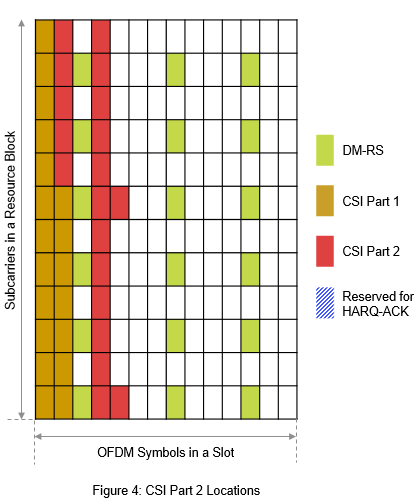Step 4: Map the coded UL-SCH data bits.

This figure shows how the UL-SCH data is mapped to the remaining locations in the grid.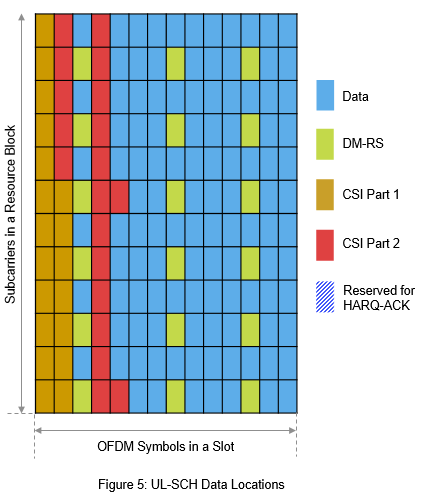Step 5: When the number of HARQ-ACK bits is less than or equal to 2, map the coded HARQ-ACK bits.

The HARQ-ACK bits are mapped within the reserved HARQ-ACK locations in a distributed pattern. This figure indicates the HARQ-ACK mapping to the grid. The HARQ-ACK punctures the CSI part 2 that occupied the reserved HARQ-ACK locations.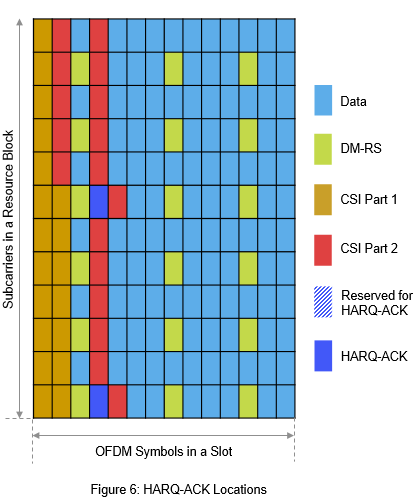Step 6: Form the codeword by reading the bits frequency first and time next approach at each RE other than DM-RS REs.

Use the nrULSCHMultiplex function to get the codeword, performing the steps 1 to 6.

% Set the payload size of the HARQ-ACK to a value less than or equal to 2
oack = 1; % Number of HARQ-ACK bits

% Get the UL-SCH coding information
info = nrULSCHInfo(pusch,tcr,tbs,oack,ocsi1,ocsi2);

% Set random coded UL-SCH, HARQ-ACK, CSI part 1, and CSI part 2 bits
culsch = randi([0 1],info.GULSCH,1);
cack = randi([0 1],info.GACK,1);
ccsi1 = randi([0 1],info.GCSI1,1);
ccsi2 = randi([0 1],info.GCSI2,1);

% Get the codeword and locations of each type (data and UCI)
[cw,indInfo] = nrULSCHMultiplex(pusch,tcr,tbs,culsch,cack,ccsi1,ccsi2);

% Create and plot the output grid for the first layer with predefined symbol
% values for the different types
createAndPlotGrid(carrier,pusch,cw,indInfo)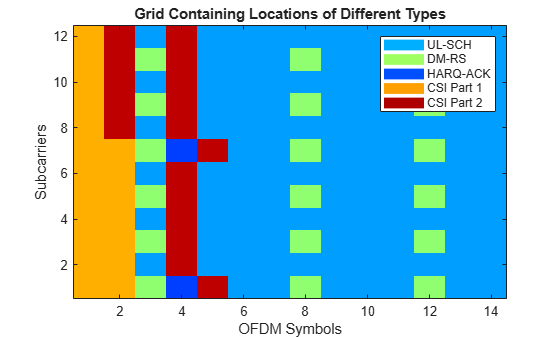Case 2: Number of HARQ-ACK Bits Greater Than 2

For the same configuration setup mentioned in case 1, change the number of HARQ-ACK bits from 1 to 3. For this setup, the number of coded HARQ-ACK bits is 6, the number of coded CSI part 1 and part 2 bits is 19 each, and the number of coded UL-SCH bits is 106.

Step 1: When the number of HARQ-ACK bits is less than or equal to 2, find the reserved HARQ-ACK locations and mark them on the grid.

Because the number of HARQ-ACK bits is greater than 2 in this case, skip this step.

Step 2: Map the coded HARQ-ACK bits.

The HARQ-ACK is mapped to the REs in the OFDM symbol that is available after the first consecutive DM-RS OFDM symbols. The number of REs required for HARQ-ACK is 6. Because this value is not greater than half of the number of REs available for UCI transmission, the mapping of the HARQ-ACK is distributed as shown in this figure.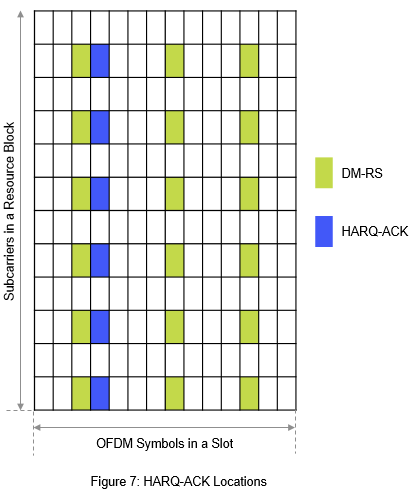Step 3: Map the coded CSI part 1 and coded CSI part 2 bits similar to case 1.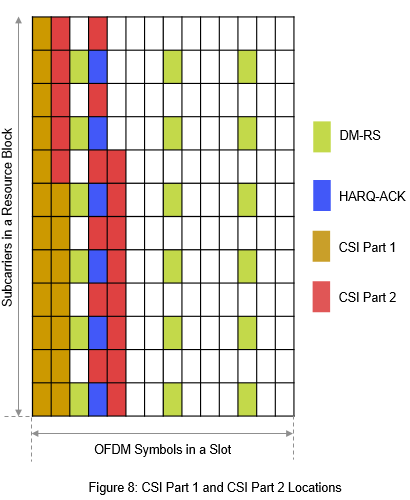Step 4: Map the coded UL-SCH data bits similar to case 1.Step 5: When the number of HARQ-ACK bits is less than or equal to 2, map the coded HARQ-ACK bits.

Because the number of HARQ-ACK bits is greater than 2 in this case, skip this step.

Step 6: Form the codeword.

% Set the payload size of the HARQ-ACK bits to a value greater than 2
oack = 3; % Number of HARQ-ACK bits

% Get the UL-SCH coding information
info = nrULSCHInfo(pusch,tcr,tbs,oack,ocsi1,ocsi2);

% Set random coded UL-SCH, HARQ-ACK, CSI part 1, and CSI part 2 bits
culsch = randi([0 1],info.GULSCH,1);
cack = randi([0 1],info.GACK,1);
ccsi1 = randi([0 1],info.GCSI1,1);
ccsi2 = randi([0 1],info.GCSI2,1);

% Get the codeword and locations of each type (data and UCI)
[cw,indInfo] = nrULSCHMultiplex(pusch,tcr,tbs,culsch,cack,ccsi1,ccsi2);

% Create and plot the output grid for the first layer with predefined symbol
% values for the different types
createAndPlotGrid(carrier,pusch,cw,indInfo)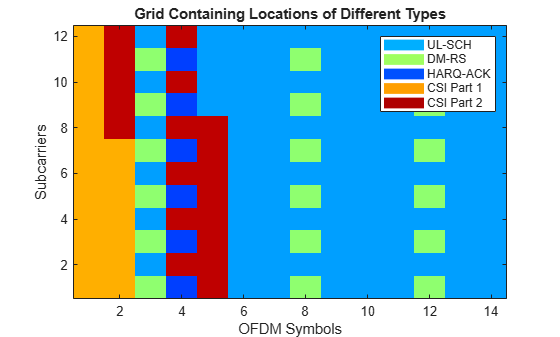Further Exploration

Change the different parameters affecting the time or frequency allocation of the PUSCH and the number of payload bits of each UCI type to vary the RE positions of the UCI types.

Enable PT-RS to vary the bit capacities of the UCI types in the codeword.

Enable intra-slot frequency hopping to observe the mapping of different UCI types in each hop.

This example shows how to generate the bit capacities of respective UCI types and perform multiplexing to generate a codeword associated with a PUSCH. The example highlights the different steps involved in multiplexing when the number of HARQ-ACK bits is less than or equal to 2 and greater than 2.

References

1. 3GPP TS 38.212. "NR; Multiplexing and channel coding (Release 15)." 3rd Generation Partnership Project; Technical Specification Group Radio Access Network.

Local Function

function createAndPlotGrid(carrier,pusch,cw,indInfo)

% Initialize the grid
qm = strcmpi(pusch.Modulation,{'pi/2-BPSK','QPSK','16QAM','64QAM','256QAM'})*[1 2 4 6 8]';
gridOFDM = complex(zeros([carrier.NSizeGrid*12 carrier.SymbolsPerSlot double(pusch.NumLayers)*qm]));

% Specify predefined values for each entity to get the desired visualization
chpLevel = struct;
chpLevel.PUSCH = 0.6;
chpLevel.DMRS = 1.1;
chpLevel.ACK = 0.4;
chpLevel.CSI1 = 1.5;
chpLevel.CSI2 = 2;

% Assign the codeword with predefined values
cw1 = zeros(size(cw));
cw1(indInfo.ULSCHIndices) = chpLevel.PUSCH;
cw1(indInfo.ACKIndices) = chpLevel.ACK ;
cw1(indInfo.CSI1Indices) = chpLevel.CSI1 ;
cw1(indInfo.CSI2Indices) = chpLevel.CSI2 ;

% Legend labels for the plot
labelStr = {'UL-SCH','DM-RS','HARQ-ACK','CSI Part 1','CSI Part 2'};

% Get the PUSCH and DM-RS indices
puschIndices = nrPUSCHIndices(carrier,pusch);
dmrsIndices = nrPUSCHDMRSIndices(carrier,pusch);

% Get the PT-RS indices
if pusch.EnablePTRS
labelStr{end+1} = 'PT-RS';
chpLevel.PTRS = 1.6;
ptrsIndices = nrPUSCHPTRSIndices(carrier,pusch);
end

% Map the DM-RS, PT-RS, and PUSCH indices to the grid
[~,puschExtInd] = nrExtractResources(puschIndices,gridOFDM);
gridOFDM(dmrsIndices) = chpLevel.DMRS;
if pusch.TransformPrecoding
% DFT-s-OFDM
titleStr = "Projections of Different Types Before Transform Precoding";
cwLen = zeros(size(puschIndices,1),pusch.NumLayers*qm);
if pusch.EnablePTRS
cwLen(ptrsIndices) = chpLevel.PTRS;
end
cwLen(cwLen==0) = cw1;
gridOFDM(reshape(puschExtInd',[],1)) = cwLen;
else
% CP-OFDM
titleStr = "Grid Containing Locations of Different Types";
if pusch.EnablePTRS
gridOFDM(ptrsIndices) =  chpLevel.PTRS;
end
gridOFDM(reshape(puschExtInd',[],1)) = cw1;
end

% Plot the grid
figure
map = jet(64);
cscaling = 30;
im = image(1:size(gridOFDM,2),1:size(gridOFDM,1),cscaling*abs(gridOFDM(:,:,1)));
colormap(im.Parent,map)

% Add a legend to the image
chpval = struct2cell(chpLevel);
clevels = cscaling*[chpval{:}];
N = length(clevels);
L = line(ones(N),ones(N), 'LineWidth',8); % Generate lines
% Index the color map and associate the selected colors with the lines
set(L,{'color'},mat2cell(map(min(1+clevels,length(map)),:),ones(1,N),3)) % Set the colors according to the map
% Create the legend
legend(labelStr{:})
axis xy
ylabel('Subcarriers')
xlabel('OFDM Symbols')
title(titleStr)

end